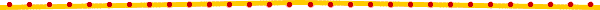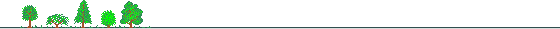# Chapter 1Introduction## Brief Description of the Energy Statistical Databank

This databank consists of 4 parts with bank names OECDR, OECDC, OECDO and ENG:

 (1) OECDR: 3,120 time series, including Taiwan's energy data listed in "Taiwan Energy Balance Table -- OECD Energy Statistical Format (in Original Units)", Energy Statistics Monthly, Chapter I, Table 13; (2) OECDC: 3,200 time series, including Taiwan's energy data listed in "Taiwan Energy Balance Table -- OECD Energy Statistical Format (in Calorific Units)", Energy Statistics Monthly, Chapter I, Table 14; (3) OECDO: 3,200 time series, including Taiwan's energy data listed in "Taiwan Energy Balance Table -- OECD Energy Statistical Format (in Oil Equivalent Units)", Energy Statistics Monthly, Chapter I, Table 15; (4) ENG: 188 time series, including Taiwan's energy data published in Energy Statistics Monthly, Chapter III, Table 1-19, and Chapter IV, Tables 1.

Data published in: Energy Statistics Monthly, Chapter I, Tables 13, 14, 15, Chapter III, Tables 1-19, and Chapter IV, Tables 1.

Data released by: Energy Commission, Ministry of Economic Affairs.

Data updated monthly.

## 1.2 Composition of Retrieval Codes

In Chapter 2, we will list all retrieval codes of ENG (i.e. part (4) described above) according to the Table order in the publication. In Chapter 3, the same retrieval codes are listed alphabetically.

In this section, we will explain the retrieval codes for OECDR, OECDC and OECDO (i.e. parts (1) (2) (3) described above).

The retrieval codes are composed as:

 Initial Letters + column number + @ + row number (OR,OC or OO)

There are 3 sets of initial letters: OR for all data in OECDR, OC for all data in OECDC, and OO for all data in OECDO. The column number and the row number refer to the standard column and row numbers used in OECD Energy Statistical Format.

Thus, for example, OR1@36 is the retrieval code for "Consumption of Coal and Its Products by the Paper Industry (in the Unit of Metric Tons Coal Equivalent)" -- "OR" are the standard initial letters for all energy data in the original units (i.e. in the bank OECDR); "1" is the column number in OECD Format standing for "Coal and Its Product"; "36" is the row number in OECD Format standing for the "Paper Industry".

Likewise, OC1@36 is the retrieval code for "Consumption of Coal and Its Products by the Paper Industry (in Calorific Units)" which is a time series in the bank OECDC; OO1@36 is the retrieval code for the same data in Oil Equivalent units which is a time series in OECDO.

You can easily construct the retrieval codes for the data you need once you know the column and row numbers in the OECD Energy Statistical Format.

For your reference, Tables 13, 14, 15 of Chapter I of the Energy Statistics Monthly (which show the Taiwan Energy Balance Table in OECD Energy Statistical Format) are reprinted here. To view them, please click:

Table 13. Taiwan Energy Balance Table - OECD Energy Statistical Format (in Original Units)

Table 14. Taiwan Energy Balance Table - OECD Energy Statistical Format (in Calorific Units)

Table 15. Taiwan Energy Balance Table - OECD Energy Statistical Format (in Oil Equivalent Units)